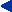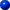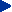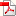This chapter in pdf-format: www.researchgate.net/publication/321224354II

Bioelectric Sources and Conductors
and Their Modeling

A practical way to investigate the function of living organisms is to construct a simple model that follows their operation with reasonable accuracy. Thus, to investigate the function of bioelectric sources and conductors, we need to construct models that accurately describe the bioelectric behavior of the tissue they represent and that can be mathematically analyzed.Chapter 7 of Part II first characterizes the nature of bioelectric sources and conductors. It points out that in contrast to electronic circuits, in which the electric properties of the components are concentrated, the biological organs are distributed volume sources and volume conductors. The standard equations describing the electric field of a volume source in a volume conductor are derived. The electric properties of the human body as a volume conductor are then characterized. This is followed by an introductory discussion of modeling of the biological volume sources and volume conductors. The fundamental concepts of forward and inverse problems are then defined and their solvability is discussed.Chapter 8 provides a detailed theoretical discussion of various source-field models and their mathematical basis. Chapter 9 follows with a discussion of a model of the biological tissue as a volume conductor. This is called the bidomain model.Chapter 10 further explores the modeling of biological sources in regard to electronic neuron models. The bioelectric behavior of neural cells and the electric concepts used in this discussion are further exemplified with electronic circuits. The discussion on electronic neuron models may also serve as a basic introduction to neurocomputers, which are a fascinating example of applying biological principles to technological systems. That topic is, however, far beyond the scope of this book.